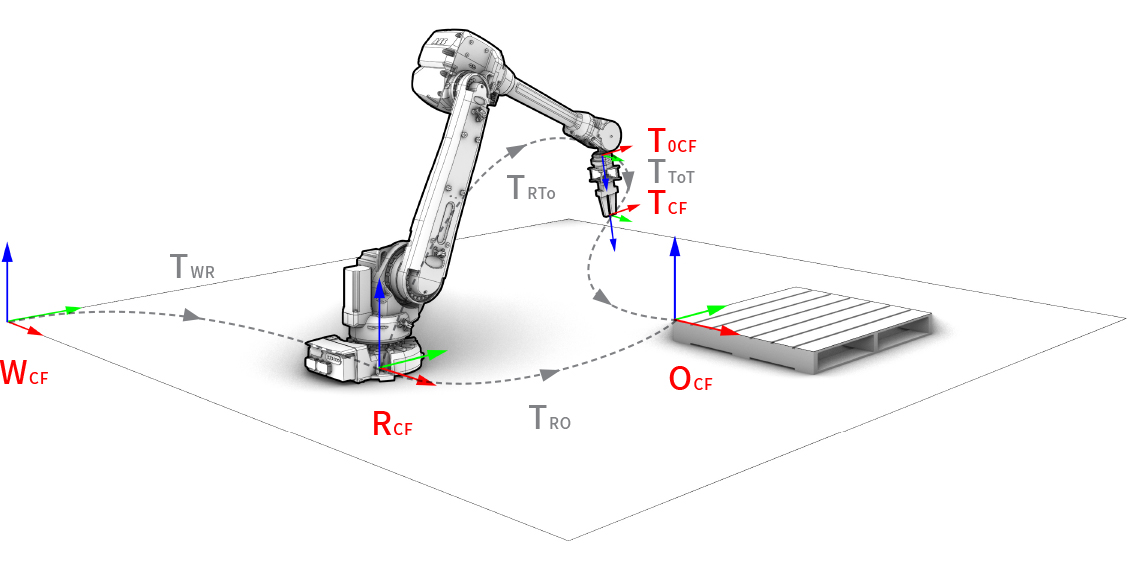# 1.2. Coordinate frames

Developers and users of robot drivers, models, and libraries need a shared convention for coordinate frames in order to better integrate and re-use software components. To plan robotic fabrication processes, the definition of robotic targets has to follow the convention of a specific relationship between coordinate frames, for example:

• World coordinate frame (WCF)

• Robot coordinate frame (RCF)

• Tool0 coordinate frame (T0CF)

• Tool coordinate frame (TCF)

• Object coordinate frame (OCF)Coordinate frame convention of a robotic setup.

To describe these coordinate frames, the compas.geometry.Frame class of the COMPAS framework is used.

## 1.2.1. World coordinate frame (WCF)

The world coordinate frame (WCF) has its origin on a fixed position with its Z-axis pointing upwards (= map in ROS convention). The WCF is important for processes that use several robots which share one space, robots with external axes, and mobile robots. By default, the WCF coincides with the robot coordinate system (RCF).

## 1.2.2. Robot coordinate frame (RCF)

The robot coordinate frame (RCF) (= base_link in ROS convention) has its origin in the base of the robot and is the reference system for the mechanical buildup of the robot. It must be defined in reference to the fixed coordinate frame WCF.

## 1.2.3. Tool0 coordinate frame (T0CF)

The tool0 coordinate frame (T0CF) has its origin at the tip of last link of the robot. It is dependent on the RCF.

## 1.2.4. Tool coordinate frame (TCF)

The tool coordinate frame (TCF) has its origin at the tip of the tool (Tool Center Point: TCP). It must be defined in reference to the T0CF.

## 1.2.5. Object coordinate frame (OCF)

The object coordinate frame (OCF) corresponds to the work object or the built structure. It defines the location of the work object in relation to the world coordinate frame (WCF).

## 1.2.6. Example

Here is a simple example of how to express the frame of an object that is defined in the world coordinate frame in the robot’s own coordinate frame before sending it as a target pose to the robot.

from compas_fab.robots import Robot

from compas.robots import RobotModel
from compas.robots import Joint

from compas.geometry import Frame
from compas.geometry import Transformation

robot_model = RobotModel('ur5',
joints=[
])

print("robot model: ", robot_model)

robot = Robot(robot_model)

print("robot coordinate frame (base)", robot.get_base_frame())

point =  [6.0, 4.0, 2.0]
xaxis =  [-1.0, 0.0, 0.0]
yaxis =  [0.0, -1.0, 0.0]

frame_WCF = Frame(point, xaxis, yaxis)
print("frame in WCF", frame_WCF)

frame_RCF = robot.to_local_coords(frame_WCF)
print("frame in RCF", frame_RCF)

frame_WCF = robot.to_world_coords(frame_RCF)
print("frame in WCF", frame_WCF)